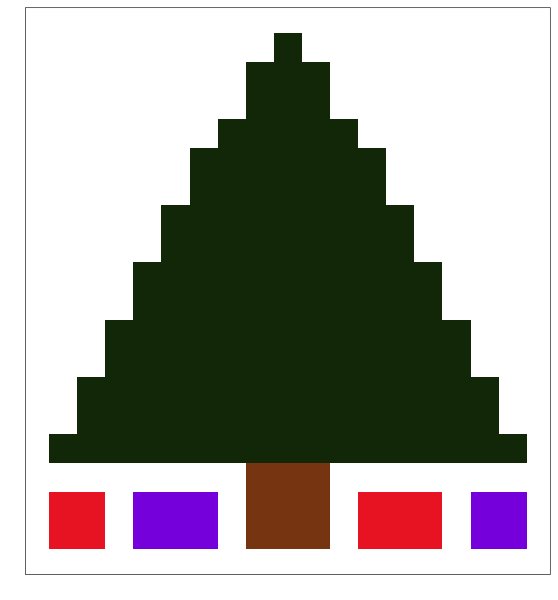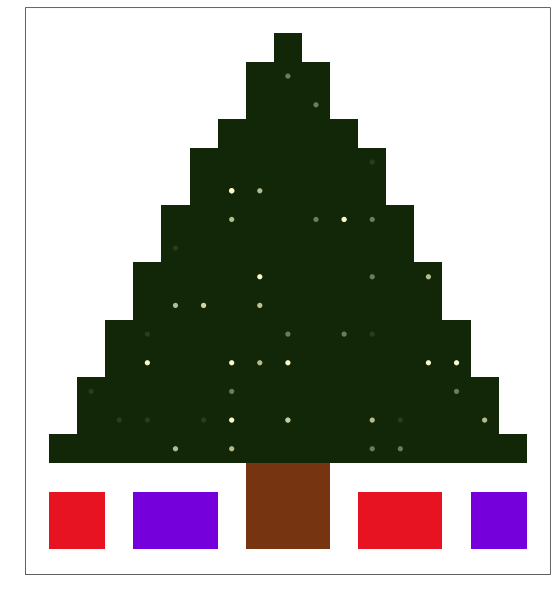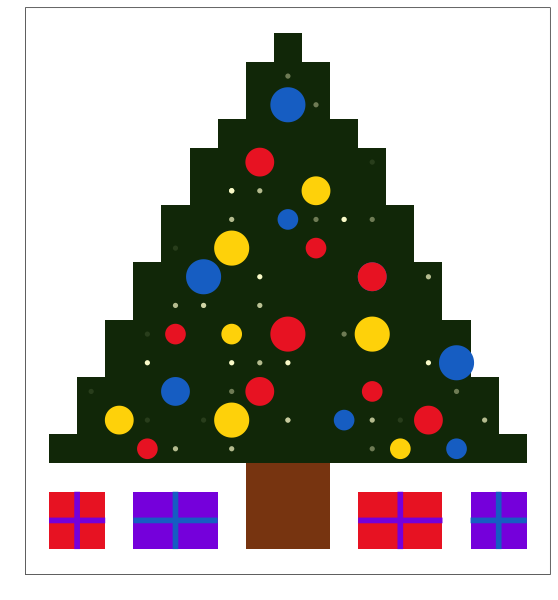# A very ggplot2 Christmas

written in

About a month ago, this classic gem popped up in my Twitter feed. For those unable to view the image, it is a Thanksgiving turkey made completely in ggplot2 by the guys over at is.R. I was so inspired by this plot I decided to see whether I could similarly draw a Christmas tree using only ggplot2:For those of you who want to jump straight to the final product, here is the code you’ll need to reproduce the chart above.

For those who want more of a step-by-step guide, I’ll talk you through how I built up each layer of the picture.

## Your base tree

In order to make the base tree, I followed pretty much the same approach used by the guys at is.R to create their turkey plot. If you have a look at their gist, you can see they created a dataframe which contained all of the parts of the turkey image assigned to different parts of the plot grid. Given that I’m not really all that artistic, I found an image of a Christmas tree that I could similarly adapt. I’ve also used the same code is.R used to plot the basic tree.

ChristmasTree <- read.csv("https://raw.githubusercontent.com/t-redactyl/Blog-posts/master/Christmas%20tree%20base%20data.csv")

library(ggplot2)

tree <- ggplot() +
geom_tile(data = ChristmasTree, aes(x = Tree.X, y = Tree.Y, fill = Tree.Colour)) +
scale_fill_identity() +
theme_bw() +
scale_x_continuous(breaks = NULL) +
scale_y_continuous(breaks = NULL) +
labs(x = "", y = "")
treegeom_tile() plots each datapoint as a solid square. They then added some formatting options: theme_bw() changes the background colour to white; scale_x_continuous(breaks = NULL) (and its y-axis counterpart) gets rid of the tickmarks and gridlines; and labs(x = "", y = "") gets rid of the axis labels.

## Hanging some lights

We can now add some lights to the tree, which I’ve done by overlaying a scatterplot on top of our base tile plot. In order to get a sort of natural looking distribution, I randomly generated x and y coordinates for each of the lights (drawing from a uniform distribution). However, given that the tree is a triangle, I needed to make sure that the lights were not generated outside the tree.

To do this, I divided the area of the tree into 6 different rectangles, and generated coordinates for the lights within each of these blocks. However, you can see that each of these occupies a different amount of area (e.g., the bottom block is much larger than the top block). In order to make sure the lights were evenly distributed across the tree, I worked out what proportion of the total area each of these rectangles roughly makes up, and used this as a multiple of the total number of lights I wanted. So for example, the bottom block makes up about 35% of the total area, versus 5% for the top block. So if I wanted 50 lights in total, I’d generate 18 lights for the bottom of the tree, and 3 for the top.

Now we have the placement of the lights sorted, let’s get them to twinkle! To do this, I created a third randomly generated variable with values from 1 to 4. We’ll use this to control the transparency of each of the dots.

Desired.Lights <- 50
Total.Lights <- sum(round(Desired.Lights * 0.35) + round(Desired.Lights * 0.20) +
round(Desired.Lights * 0.17) + round(Desired.Lights * 0.13) +
round(Desired.Lights * 0.10) + round(Desired.Lights * 0.05))

Lights <- data.frame(Lights.X = c(round(runif(round(Desired.Lights * 0.35), 4, 18), 0),
round(runif(round(Desired.Lights * 0.20), 5, 17), 0),
round(runif(round(Desired.Lights * 0.17), 6, 16), 0),
round(runif(round(Desired.Lights * 0.13), 7, 15), 0),
round(runif(round(Desired.Lights * 0.10), 8, 14), 0),
round(runif(round(Desired.Lights * 0.05), 10, 12), 0)))
Lights$Lights.Y <- c(round(runif(round(Desired.Lights * 0.35), 4, 6), 0), round(runif(round(Desired.Lights * 0.20), 7, 8), 0), round(runif(round(Desired.Lights * 0.17), 9, 10), 0), round(runif(round(Desired.Lights * 0.13), 11, 12), 0), round(runif(round(Desired.Lights * 0.10), 13, 14), 0), round(runif(round(Desired.Lights * 0.05), 15, 17), 0)) Lights$Lights.Colour <- c(round(runif(Total.Lights, 1, 4), 0))


We now add the lights to our tree by assigning it to a geom_point() command. We let ggplot2 know that the base colour of our lights is “lightgoldenrodyellow” by assigning it to the colour argument (make sure you assign it the colour argument outside aes, as these are two different arguments!), and that we want to alter the transparency of the dots by assigning Lights.Colour to the alpha argument (this time inside aes).

We also suppress the legend that has just been created by adding theme(legend.position = "none").

tree <- tree +
geom_point(data = Lights, aes(x = Lights.X, y = Lights.Y, alpha = Lights.Colour),
colour = "lightgoldenrodyellow", shape = 16) +
theme(legend.position = "none")
treeLook at that tree sparkle!

## Adding the baubles

Let’s now hang some baubles. To do this, we add yet another scatterplot (this time a weighted scatterplot, one of my favourite plot types) on top of our two existing plots. I found the random approach didn’t work so well with these, so I manually plotted the coordinates of each of these, as well as manually assigning both a colour and a size to each bauble. You can feel free to tinker with these positions and sizes on your own tree.

Baubles <- data.frame(Bauble.X = c(6, 9, 15, 17, 5, 13, 16, 7, 10, 14, 7, 9, 11,
14, 8, 14, 9, 12, 11, 12, 14, 11, 17, 10))
Baubles$Bauble.Y <- c(4, 5, 4, 4, 5, 5, 5, 6, 6, 6, 8, 8, 8, 8, 10, 10, 11, 11, 12, 13, 10, 16, 7, 14) Baubles$Bauble.Colour <- factor(c(1, 2, 2, 3, 2, 3, 1, 3, 1, 1, 1, 2, 1, 2,
3, 3, 2, 1, 3, 2, 1, 3, 3, 1))
Baubles\$Bauble.Size <- c(1, 3, 1, 1, 2, 1, 2, 2, 2, 1, 1, 1, 3, 3, 3,
2, 3, 1, 1, 2, 2, 3, 3, 2)


Now let’s add these to our tree using a weighted scatterplot command:

tree <- tree +
geom_point(data = Baubles, aes(x = Bauble.X, y = Bauble.Y,
colour = Bauble.Colour, size = Bauble.Size),
shape = 16) +
scale_colour_manual(values = c("firebrick2", "gold", "dodgerblue3")) +
scale_size_area(max_size = 12)
treeI’ve given the baubles the colours “firebrick2”, “gold” and “dodgerblue3” from the list of recognised colours, but you can of course tweak these to be anything you want, especially if you have a bit more taste than I do! The default size of the baubles was a bit small, so I’ve also increased their size using the scale_size_area() option.

## Decorating the presents

Now those presents look a little bare, right? Let’s spruce them up a little with some nice ribbons. We can do this using the geom_segment() option.

tree <- tree +
geom_segment(aes(x = 2.5, xend = 4.5, y = 1.5, yend = 1.5), colour = "blueviolet", size = 2) +
geom_segment(aes(x = 5.5, xend = 8.5, y = 1.5, yend = 1.5), colour = "dodgerblue3", size = 2) +
geom_segment(aes(x = 13.5, xend = 16.5, y = 1.5, yend = 1.5), colour = "blueviolet", size = 2) +
geom_segment(aes(x = 17.5, xend = 19.5, y = 1.5, yend = 1.5), colour = "dodgerblue3", size = 2) +
geom_segment(aes(x = 3.5, xend = 3.5, y = 0.5, yend = 2.5), colour = "blueviolet", size = 2) +
geom_segment(aes(x = 7.0, xend = 7.0, y = 0.5, yend = 2.5), colour = "dodgerblue3", size = 2) +
geom_segment(aes(x = 15.0, xend = 15.0, y = 0.5, yend = 2.5), colour = "blueviolet", size = 2) +
geom_segment(aes(x = 18.5, xend = 18.5, y = 0.5, yend = 2.5), colour = "dodgerblue3", size = 2)
treeYou can see that for the horizontal ribbons, I’ve put a range of x-coordinates but put the same value for both of the y-coordinates in order to get a straight line, and vice versa for the vertical lines. You can also alter the colour and thickness of the ribbons using the colour and size arguments respectively.

## Writing a greeting

Finally, let’s top off our tree with a festive greeting. The default fonts in R are not particularly decorative, so let’s import some extra fonts using the extrafont package (more instructions on using this package are here).

library(extrafont)
font_import()


We can now check what fonts we have using the fonts() command. Of my installed fonts “Luminari” has the most old timey feel, so let’s use this for the greeting.

tree <- tree +
annotate("text", x = 11, y = 20, label = "Merry Christmas!",
family = "Luminari", size = 12)
treeYou can see I’ve used the annotate option with the “text” argument to insert “Merry Christmas!” at coordinates (11, 20). I’ve then told ggplot2 that I want to use the Luminari font at size 12 using the family and size arguments respectively.

And there we have it! You now have the perfect Christmas greeting for the data nerd in your life. Happy Christmas!